# Data sufficiency Quiz for RRB PO & RRB CLERK : Quiz – 7

## Data sufficiency Quiz for RRB PO & RRB CLERK : Quiz – 7

Data Sufficiency is a vital piece of almost all competitive exams, so you can’t take the risk of leaving out it. A considerable lot of the competitors face trouble in Data Sufficiency Questions. If you practice more and more questions of Data Sufficiency Quiz, it will turn out to be simple for you. Thus, we ‘AmbitiousBaba’ are giving you Data Sufficiency Quiz to support your devising for upcoming exams like banking, Insurance, SSC, and Railway. This Data Sufficiency Quiz is totally FREE for the candidates. This Data Sufficiency Quiz is based on latest or updated exam pattern.

Directions (1 – 10): Each question below is followed by two or three statements A, B and C. You have to determine whether the data given in the statements are sufficient for answering the question. You should use the data and your knowledge of Mathematics to choose between the possible answers. Read the statements and Answer.

1. A sum of money Rs 2550 is to be distributed among A, B and C. What will be the share of B?
A. A’s share is 1.5 times B’s share,
B. C’s share is half the share of A and B together.
C. The share of A is Rs 340 more than B.
(a) Either A and B together or A and C together are sufficient
(b) Only A and B together are sufficient
(c) Only A and C together are sufficient
(d) All statements are required
(e) Any 2 of the 3 statements are sufficient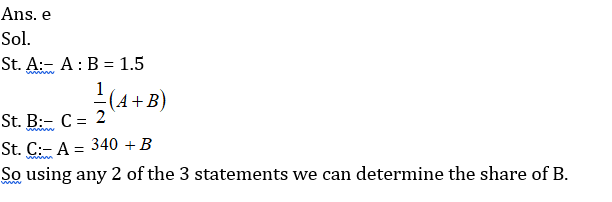2. 40 students sit in rows and columns. How many students are seated in each column if each column have same number of students?
A. The number of rows is 62.50% of the number of columns.
B. The number of rows is 5/8 of the number of columns.
C. The number of rows is less than the number of columns.
(a) Only A
(b) C and either A or B
(c) Only B
(d) Either A or B
(e) All the statements are required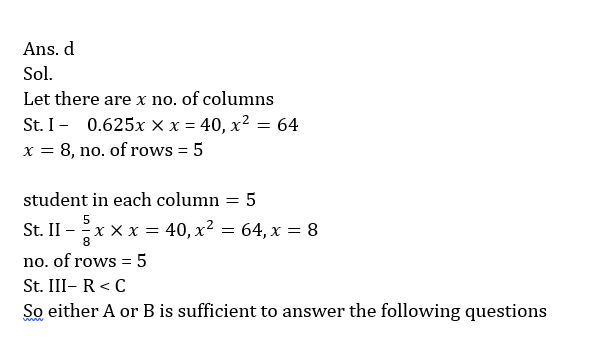3. What is the age of Ravi?
A: Four years ago, Raju was as old as Ravi is at present.
B: Rita’s present age is two times of Raju’s present age.
C: The average age of Ravi and Rita is 19 years.
(a) A is sufficient to give answer
(b) Any two statements are sufficient to give answer
(c) All of three statements are necessary to give answer
(d) Even using all the three statements answer cannot be found.
(e) Only C is sufficient.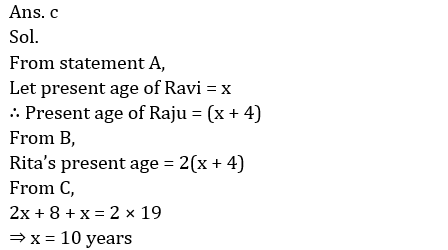4. What is the cost price of wrist watch?
A: Shopkeeper gives 20% discount on a speaker.
B: The marked price of wrist watch is 25% more than that of speaker.
C: The shopkeeper earns a profit of 10% after selling the speaker.
(a) Any two of them are sufficient
(b) All the three statements are required
(c) Only C is sufficient.
(d) Even using all statements, answer cannot be found
(e) Only A is sufficient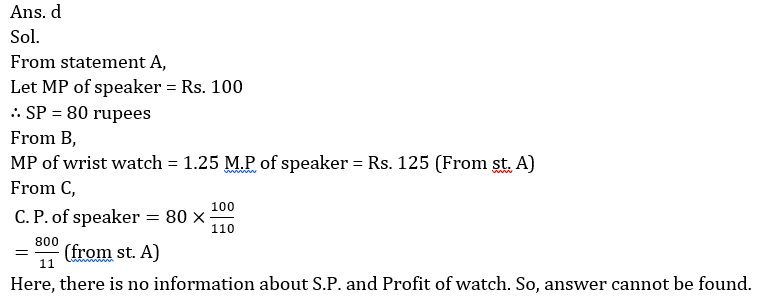5. In how many ways 2 green balls can be chosen from box ‘A’ which contains red, green and black balls?
A: Ratio of total number of balls in boxes A and B is 2 : 3 and box A contains 5 green balls.
B: Total balls in box B is 18 while ratio of red and black balls in box A is 3:4.
C: Box ‘B’ contains 5 red 7 black and 6 green balls only while ratio of red and green balls in box ‘A’ is 3:5.
(a) Statement A and either B or C are sufficient
(b) Any two statements are sufficient
(c) All the three statements are required
(d) Statement B and either A or C are sufficient
(e) None of these
Ans. e
Sol.
With help of statement A only, required number of ways can be found out.

6. Find the value of 8y² (y + 3x) + 8x² (x + 3y)
A) Product of x and y is equal to 6
B) Sum of inverse of x and y is equals to 5/6
(a) if the Statement ‘A’ alone is sufficient to answer the question but the Statement ‘B’ alone is not sufficient
(b) if the Statement ‘B’ alone is sufficient to answer the question but the Statement ‘A’ alone is not sufficient
(c) if both Statement ‘A’ and ‘B’ together are needed to answer the question
(d) if either the Statement ‘A’ alone or Statement ‘B’ alone is sufficient to answer the question
(e) if you cannot get the answer from both the Statements together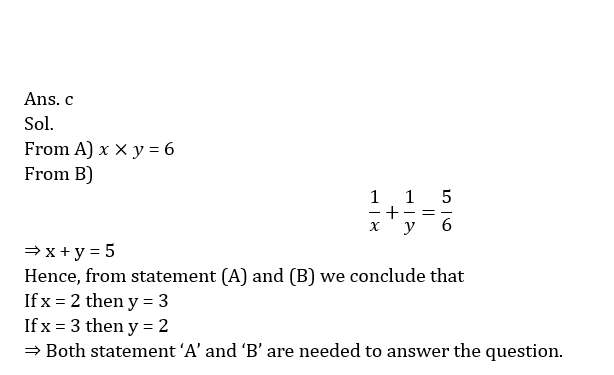7. If X, Y and Z together can complete the work in 8 days, find in how many days Y alone can complete the work?
A) X and Y together can complete the work in 12 days while Z is 25% less efficient than ‘X’.
B) Z is 50% more efficient than ‘Y’ who is 100% less efficient than ‘X’.
(a) if the Statement ‘A’ alone is sufficient to answer the question but the Statement ‘B’ alone is not sufficient
(b) if the Statement ‘B’ alone is sufficient to answer the question but the Statement ‘A’ alone is not sufficient
(c) if both Statement ‘A’ and ‘B’ together are needed to answer the question
(d) if either the Statement ‘A’ alone or Statement ‘B’ alone is sufficient to answer the question
(e) if you cannot get the answer from both the Statements together8. What will be the speed of boat if A is the starting point and B is the destination point?
A) Man go to a point B and come back to initial point A in total 12 hours while the ratio of speed of boat to speed of stream is 3 : 2.
B) Man reached at mid-point of A and B in 5 hour and then go to point B and come back to mid-point of A and B in total 6 hours while speed of stream is 10 km/hr.
(a) if the Statement ‘A’ alone is sufficient to answer the question but the Statement ‘B’ alone is not sufficient
(b) if the Statement ‘B’ alone is sufficient to answer the question but the Statement ‘A’ alone is not sufficient
(c) if both Statement ‘A’ and ‘B’ together are needed to answer the question
(d) if either the Statement ‘A’ alone or Statement ‘B’ alone is sufficient to answer the question
(e) if you cannot get the answer from both the Statements together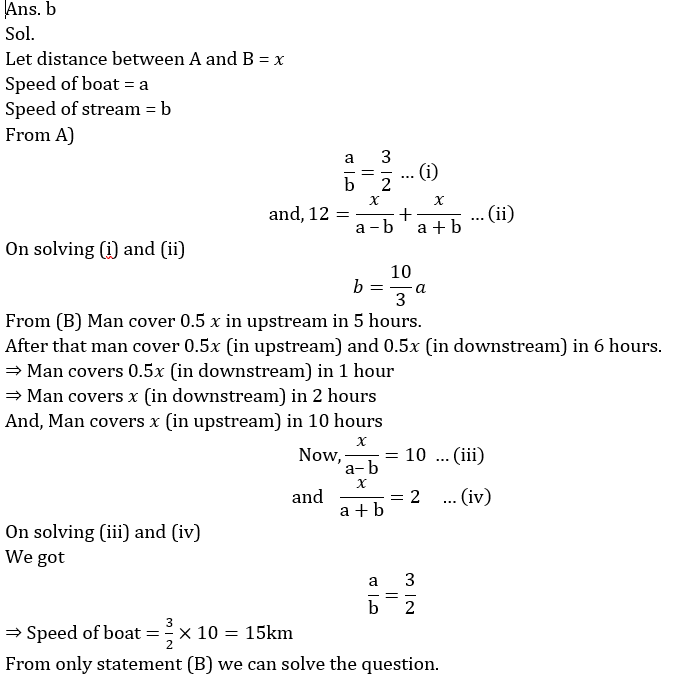9. Three numbers are co-prime to each other. Find the sum of these numbers?
A) Product of first two number is 286 while the product of last two numbers is 470.
B) Sum of first two number is 35 while the sum of last two number is 57.
(a) if the Statement ‘A’ alone is sufficient to answer the question but the Statement ‘B’ alone is not sufficient
(b) if the Statement ‘B’ alone is sufficient to answer the question but the Statement ‘A’ alone is not sufficient
(c) if both Statement ‘A’ and ‘B’ together are needed to answer the question
(d) if either the Statement ‘A’ alone or Statement ‘B’ alone is sufficient to answer the question
(e) if you cannot get the answer from both the Statements together10. Find the sum of digits of given two-digit number?
A) If we interchange the numbers then the new number formed is greater than the original number by 9.
B) If we interchange the number then product of new number and original number is 1462.
(a) if the Statement ‘A’ alone is sufficient to answer the question but the Statement ‘B’ alone is not sufficient
(b) if the Statement ‘B’ alone is sufficient to answer the question but the Statement ‘A’ alone is not sufficient
(c) if both Statement ‘A’ and ‘B’ together are needed to answer the question
(d) if either the Statement ‘A’ alone or Statement ‘B’ alone is sufficient to answer the question
(e) if you cannot get the answer from both the Statements together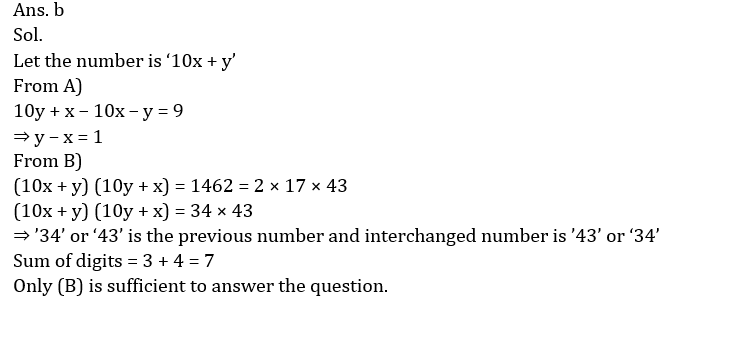#### Attempt Quantitative Aptitude Topic Wise Online Test Series

Recommended PDF’s for:

#### Most important PDF’s for Bank, SSC, Railway and Other Government Exam : Download PDF Now

AATMA-NIRBHAR Series- Static GK/Awareness Practice Ebook PDF Get PDF here
The Banking Awareness 500 MCQs E-book| Bilingual (Hindi + English) Get PDF here
AATMA-NIRBHAR Series- Banking Awareness Practice Ebook PDF Get PDF here
Computer Awareness Capsule 2.O Get PDF here
AATMA-NIRBHAR Series Quantitative Aptitude Topic-Wise PDF Get PDF here
AATMA-NIRBHAR Series Reasoning Topic-Wise PDF Get PDF Here
Memory Based Puzzle E-book | 2016-19 Exams Covered Get PDF here
Caselet Data Interpretation 200 Questions Get PDF here
Puzzle & Seating Arrangement E-Book for BANK PO MAINS (Vol-1) Get PDF here
ARITHMETIC DATA INTERPRETATION 2.O E-book Get PDF here
3